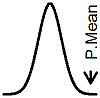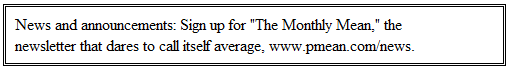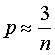Updated: Confidence interval with zero events (created 2001-01-19, updated 2010-07-07).Dear Professor Mean, I was working with a colleague on some confidence intervals for the probability of an adverse event during several different types of operations. One of the proportions was zero, since the event never occurred. My friend computed a confidence interval and it went from zero to zero. I told him that this couldn't be right and computing a confidence interval with zero events is impossible. Isn't that right? -- Killjoy Karlina

Dear Killjoy,

You have a confidence interval that goes from zero to zero?  It reminds me of that Gershwin tune "I've got plenty of nothing."

Your friend is using the traditional formula for a confidence interval which looks something likeThe traditional confidence interval formula doesn't work here, because it relies on a normal approximation. When you have a reasonable mixture of events and non-events, then this approximation is fine. But if the number of events or non-events is small (say less than five), then the normal approximation is a lousy approximation.

So your friend is wrong if he believes in his zero to zero confidence interval.

On the other hand, you can still compute a confidence interval for this data. The simplest formula uses the rule of three. If you observe n patients, and none of these patients have an adverse event, then a 95% confidence interval for the probability of an adverse event goes from zero to 3/n.

There are more precise formulas that rely on the binomial distribution, but the rule of three is a pretty good approximation, even if the number of patients studied is small.

More details

The rule of three is an interesting application of probability. Let's suppose that we are following 14 chemotherapy patients, and we notice that none of them develop testicular cancer, which is a possible side effect of the treatment. Can these 14 boys tell us something about the risk of testicular cancer?

We can start by asking ourselves what the probability of zero events out of 14 would be if the risk of testicular cancer were 3%. A standard formula in Statistics tells us that if we observe k events, the events are independent, and the probability of each event is p, then the probability of all k events occurring simultaneously is p^k ( p raised to the kth power).

The probability of no testicular cancer in a single boy is .97, so the probability for 14 boys would by .97 raised to the 14th power which works out to be around 0.65. This tells us that an adverse event rate of 3% is REASONABLY CONSISTENT with a data set that has zero adverse events out of 14.

Let's try a different scenario. Let's assume the risk of testicular cancer in any single boy would be 30%. This means the probability of seeing no events in 14 boys would be .70 raised to the 14th power which equals 0.0068. This is such a small probability that we would have to say that no events out of 14 boys is INCONSISTENT with the assumption of a 30% adverse event rate.

We get a 95% confidence interval by finding the range of assumed adverse event rates which lead to a probability of 5% or more. We can express this with the following algebraic equationwhere "p" is the assumed probability of an adverse event and "n" is the number of patients for whom we failed to notice any adverse events. Using high school algebra, we can show that this equation is equivalent towhere "ln" represents the natural logarithm. I bet you've already forgotten all your high school algebra, haven't you? Well if you have, you won't like the next step either. Do you remember Taylor's theorem from Calculus? We can use Taylor's theorem to show the following approximationfor p close to zero. This, plus the fact that the natural log of.05 is roughly -3, simplifies our equation toorSo zero to 3/n is an approximate 95% confidence interval for a data set where we observed 0 events in n patients. This approximation works very well, even if we only have a few patients.

Example

Let's refer again to the example of testicular cancer. We observe 14 boys undergoing chemotherapy and none of them develop testicular cancer. What is a 95% confidence interval for the rate of testicular cancer in boys who have chemotherapy?

Using the rule of 3, we have the 95% confidence interval going up to 3/14=0.214. So we are 95% confident that the rate of testicular cancer for all boys having chemotherapy is 21% or less.

Let's compare this to an exact calculation of the confidence interval. The following is an excerpt from the output of a computer program, StatXact, that computes exact confidence intervals for any sample size.

Number of Trials =14 Number of Successes =0
Point Estimation of PI = 0.0000
95.00% Confidence Interval for PI = ( 0.0000 , 0.2316)

We see that the exact confidence interval extends up to 23%. So even with only 14 patients, our approximate confidence interval based on the rule of 3 is not off by too much. As the sample size increases, this approximation gets better and better.

Summary

Killjoy Karlina is criticizing a colleague for computing a confidence interval for a sample proportion that was zero. This confidence interval used the classical formula and was clearly invalid since it went from zero to zero. Professor Mean explains the rule of three: when you have zero event out of n, a 95% upper confidence limit for the true proportion is 3/n. This rule can help you plan sample sizes for events that are very rare.